#Radioactive DecayMany radioactive materials disintegrate at a rate proportional to the amount present. For example, if X is the radioactive material and Q(t) is the amount present at time t, then the rate of change of Q(t) with respect to time t is given by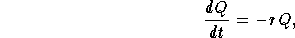where r is a positive constant (r>0). Let us call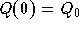the initial quantity of the material X, then we have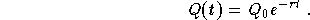Clearly, in order to determine Q(t) we need to find the constant r. This can be done using what is called the half-life T of the material X. The half-life is the time span needed to disintegrate half of the material. So, we have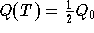. An easy calculation gives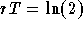. Therefore, if we know T, we can get r and vice-versa. Many chemistry text-books contain the half-life of some important radioactive materials. For example, the half-life of Carbon-14 is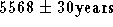. Therefore, the constant r associated with Carbon-14 is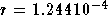. As a side note, Carbon-14 is an important tool in the archeological research known as radiocarbon dating.

Example: A radioactive isotope has a half-life of 16 days. You wish to have 30 g at the end of 30 days. How much radioisotope should you start with?

Solution: Since the half-life is given in days we will measure time in days. Let Q(t) be the amount present at time t and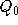the amount we are looking for (the initial amount). We know that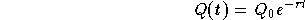,

where r is a constant. We use the half-life T to determine r. Indeed, we have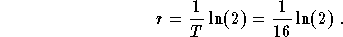Hence, since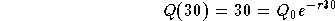,

we get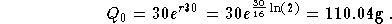[Differential Equations] [First Order D.E.]
[Geometry] [Algebra] [Trigonometry ]
[Calculus] [Complex Variables] [Matrix Algebra]S.O.S MATHematics home page

Do you need more help? Please post your question on our S.O.S. Mathematics CyberBoard.